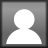# Math Problem Solving Part 4: Equal Groups Story Problems

Are you ready for a way to help students solve multiplication and division word problems? I have developed a template that will help students record the given facts and think through the process involving equal groups. There are 3 basic types of equal groups problems:• # of groups and # in each group are known
• # of groups and total are known
• # in each group and total are known

Pictured here is an illustration of the strategy which can be used for multiplication and division problems. Get my FREE packet right here: Equal groups strategy with template.

One of the most important steps I recommend when working with equal groups problems is for students to brainstorm things that typically come in equal groups. There is a great book titled “What Comes in 2’s, 3’s, and 4’s” which can set the stage. It’s a picture book, but helpful to get kids’ brains warmed up.  Here are some visuals I made to illustrate the point. Click here for your FREE copy. They are included in this set of Equal groups pictures and list template

Kids may need your help to think of things to add to the list. See some hints below. This template and a full list is included in the above FREE download.Now, here is a resource I have been working on all week! I am so excited to bring it to you!! This contains word problems on task cards in which students can practice using the circles / square template to solve equal groups problems.  You can get it FREE right here: Multiplication and Division task cards

These cards focus more on equal groups problems using the basic multiplication / division facts. I will be working on problems with larger numbers in the near future – so stay tuned!

One of the main points I want to make with this strategy is this:

• When the # of groups and # in each group is known (both circles), then multiply to find the product / total.• When the # of groups and the total are known (circle and square), then you can solve by thinking of the missing factor or by dividing.  You want students to see the relationship between multiplication and division. When students ask themselves, “How many 5’s are in 20?” they are processing this:  5 x ___ = 20 and this:  20 ÷ 5 = 4• When the # in each group and the total are known (circle and square), then the way to solve is the same as above.So my suggestion is not to give a blanket statement such as this is a “division” or a “multiplication” problem, but refer to them as an “equal groups” problem.  This is because these can be approached in different ways . . . it depends on what two pieces of information are given in the problem.

I will conclude my series of story problems with a strategy for comparison problems involving equal groups in a future post. (Joe has 4 cookies. Manuel has 3 times more than Joe.).  Let me know your thoughts if you give these strategies a try. If I already visit your school, I’d be glad to help you with this!

## 2 thoughts on “Math Problem Solving Part 4: Equal Groups Story Problems”

1.Barbara Keck

Thank you for the templates; I hope to use them soon.

2.Cindy

Thank you! Hope these help!